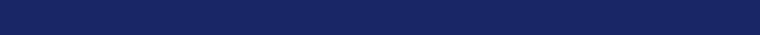MedFriendly®Alpha
1. The 1st letter of the Greek alphabet, which is used as
a means by which to classify things in many sciences.
The most common examples are listed below.

2. An abbreviation for alpha particle. An alpha particle is a
particle containing two neutrons and protons, each with a
positive charge. Protons are positively charged particles
whereas neutrons are particles with no electric charge.

FEATURED BOOK: Stedman's Medical Abbreviations,
Acronyms, and Symbols

The Greek letter alpha

3. In statistics, alpha is used to express the degree by which a statistical finding can be
expected to have occurred by chance. In other words, if alpha is .05, the probability that
the finding occurred by chance is 5%.

4. In chemistry, alpha is often used to denote a) the 1st in a series, b) the first in a
series of closely related compounds, c) the direction of a chemical bond away from the
viewer, d) a position immediately next to a carboxyl group, and e) an aromatic
substituent on an aliphatic chain.

A substituent is a group of molecules that replaces another in a chemical reaction. In
chemistry, aromatic means containing one or more benzene rings. Benzene is a type of
colorless, highly flammable, liquid hydrocarbon (a compound made of the elements,
hydrogen and carbon).
"Where Medical Information is Easy to Understand"™
An aliphatic chain is a sequence in which carbon atoms are joined
together in straight or branched chains. A carboxyl group is a
molecule composed of one carbon atom attached to an oxygen
atom by sharing two pairs of electrons and to a hydroxyl group by
sharing one pair of electrons.

An atom is the smallest part of a substance that can exist alone or
in combination with something else. A molecule is a combination of
atoms. An electron is a negatively charged particle that is smaller
than an atom. A hydroxyl group is a molecule that contains an
oxygen atom and a hydrogen atom connected by two pairs of
electrons.5. In chemistry, alpha is also used as the symbol for the angle of optic rotation. Optic rotation is the
change in the rotation of polarized light of a given wavelength as it travels through certain substances.
Polarization is the property of electromagentic waves (such as light) that describes the direction that the
electrical field flows perpendicular to the direction of travel. All electromagnetic waves have electric and
magnetic fields perpendicular to the direction of travel. Longitudinal waves (unlike transverse waves) do
not exhibit polarization because their waves vibrate along the direction of travel. Transverse waves
causes vibration in a perpendicular direction to its own motion. Perpendicular means a straight line at
right angles to another line. A good example would be the lines of the letter "L."

6. Alpha is also used in chemistry to distinguish one variation of a chemical compound from another.

7. The symbol for alpha is sometimes used to represent the Bunsen solubility coefficient. The Bunsen
solubility coefficient is the milliliters of gas dissolved per milliliter of liquid that is maintained at
atmosphere pressure and at a given temperature. A milliliter is one thousandth of a liter, meaning that it
takes one thousand milliliters to equal one liter. The coefficient is expressed as a volume of gas
assuming that the gas is at normal pressure, normal, temperature, and dry.

8. A type of bodily rhythm or wave. An example is an alpha wave, which is a type of electrical wave
detected in the brain during sleep.
NOTE: The spelling "alpha" is used in chemical names whereas the spelling "alfa" is used in
pharmaceutical names.